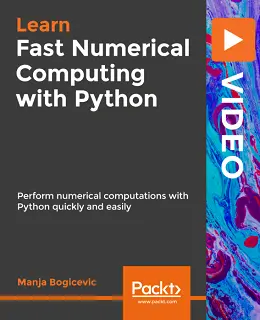# Fast Numerical Computing with Python [Video]### Fast Numerical Computing with Python [Video]

##### English | MP4 | AVC 1920×1080 | AAC 48KHz 2ch | 3h 17m | 624 MB eLearning | Skill level: All Levels

Fast Numerical Computing with Python [Video]: Get to grips with aspects of numerical computing and understand NumPy, the powerful numerical computing module, using Python!

Performing numerical computations using conventional Python methods is inefficient as complex calculations can take their toll on your system’s performance. However, NumPy, the core library for scientific computing in Python, helps keep computations fast and lets your system perform efficiently.

This course adopts a step-by-step approach where you learn through live examples. You will learn numerical computations by performing them! Gain the skills you need to become a better Python developer or data scientist. Beginning with NumPy’s arrays and functions, you’ll master linear algebra concepts, perform vector and matrix math operations, and use NumPy in Python (using quick and easy techniques) to derive numerical results faster and far more easily than with any other tool. You will understand and practice data processing and predictive modeling throughout the course.

• Develop a strong foundation for numerical computational problems
• Competently program in Python and code in Jupyter Notebooks
• Use the most significant numerical computing library available for Python: NumPy